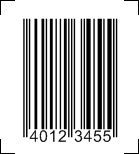# Barcode EAN 8

Code EAN 8 encodes seven digits plus a mandatory check digit, hence the name EAN 8. An EAN 8 barcode is easily recognized. It always starts and ends with two thin bars. It also has two thin bars right in the center of the symbol.

EAN 8 is used in retail, usually for chain store products which are not sold elsewhere under the same brand or name.

## Checksum for Code EAN 8

EAN 8 uses a modulo 10 checksum scheme. Starting from the right the numbers are multiplied alternating with "3" and "1" and added up. The sum is integer divided by ten, the reminder is subtracted from ten which yields the check digit.

## Example Check Digit Calculation for Code EAN 8

Using the sample barcode further below our numbers are "4012345".

Summing up: 5 * 3 + 4 * 1 + 3 * 3 + 2 * 1 + 1 * 3 + 0 * 1 + 4 * 3 = 45
Dividing: 45 / 10 = 4 Reminder 5
Check Digit: 10 - 5 = 5

## Sample Code EAN 8## Obtaining a Code EAN 8 number

EAN numbers are maintained by your country's GS1 branch, please see obtaining an EAN number for details.

## Create Code EAN 8

Softmatic BarcodePlus creates EAN 8 and exports the code as EPS (vector) and to common raster formats.

Windows PC - Softmatic® BarcodePlus (Win, V4, Windows XP or higher)